# 使用 Amazon SageMaker 构建机器学习应用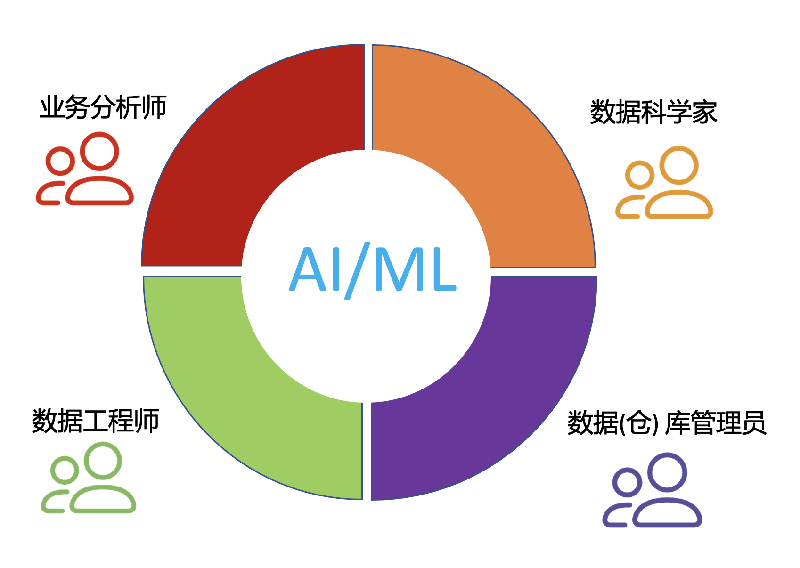## 1、介绍

Amazon SageMaker是一个端到端的机器学习平台，SageMaker支持您的模型开发全流程，从数据准备，数据处理，算法构建，模型训练，超参调优，模型部署与监控等环节，SageMaker都提供了相应的功能帮助算法工程师们专注于业务和模型本身，提高开发效率。

## 2、创建SageMaker Notebook实例

Amazon SageMaker 提供了两种方式的Jupyter Notebook，Notebook实例SageMaker Studio Notebook。不管哪种方式，SageMaker都提供了预置的开发环境，如TensorFlow，PyTorch，Numpy，Pandas等等，算法工程师无需自行安装，而且SageMaker也支持自定义环境，充分保证了灵活性。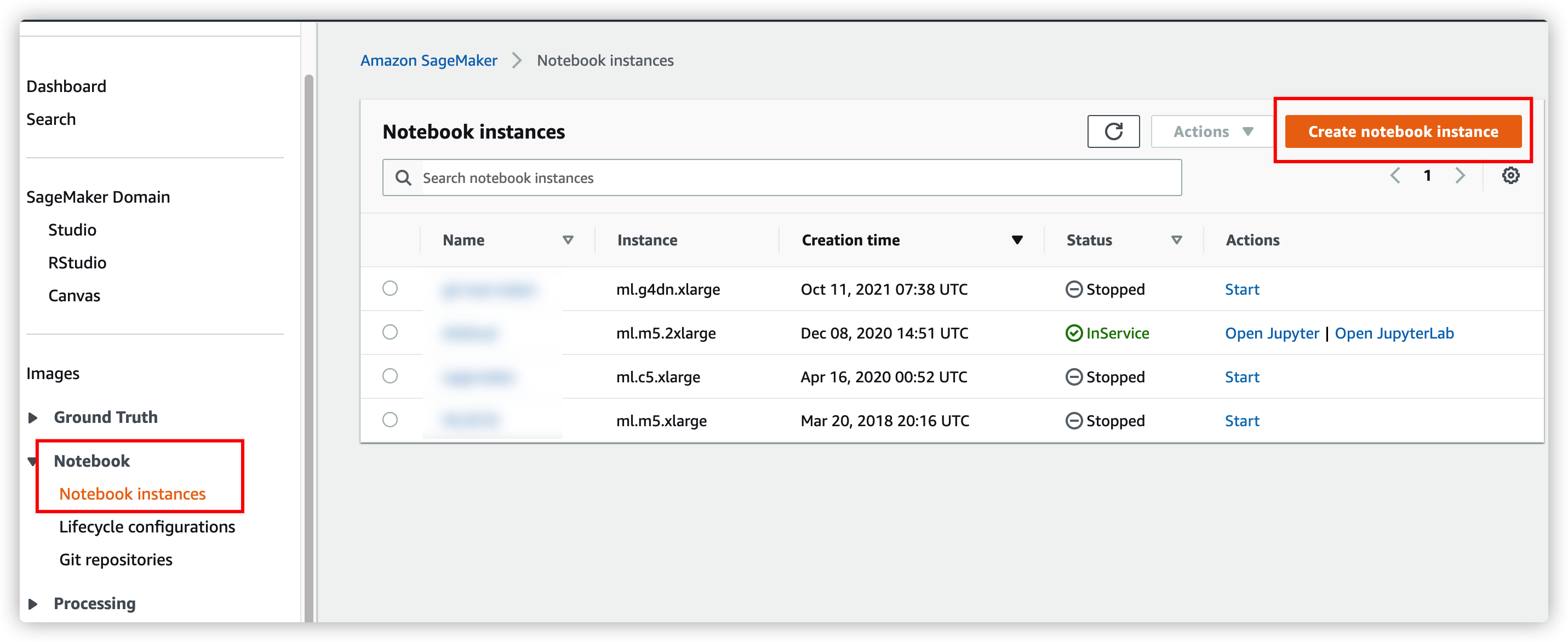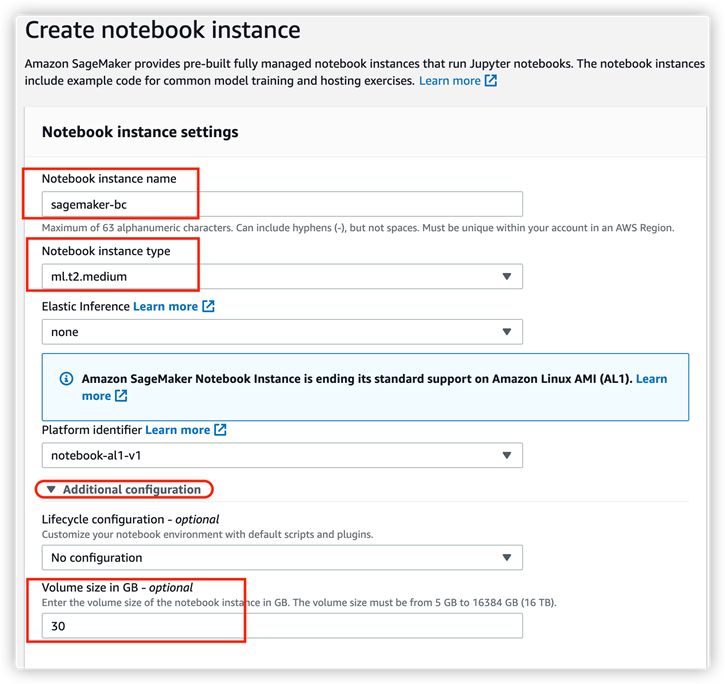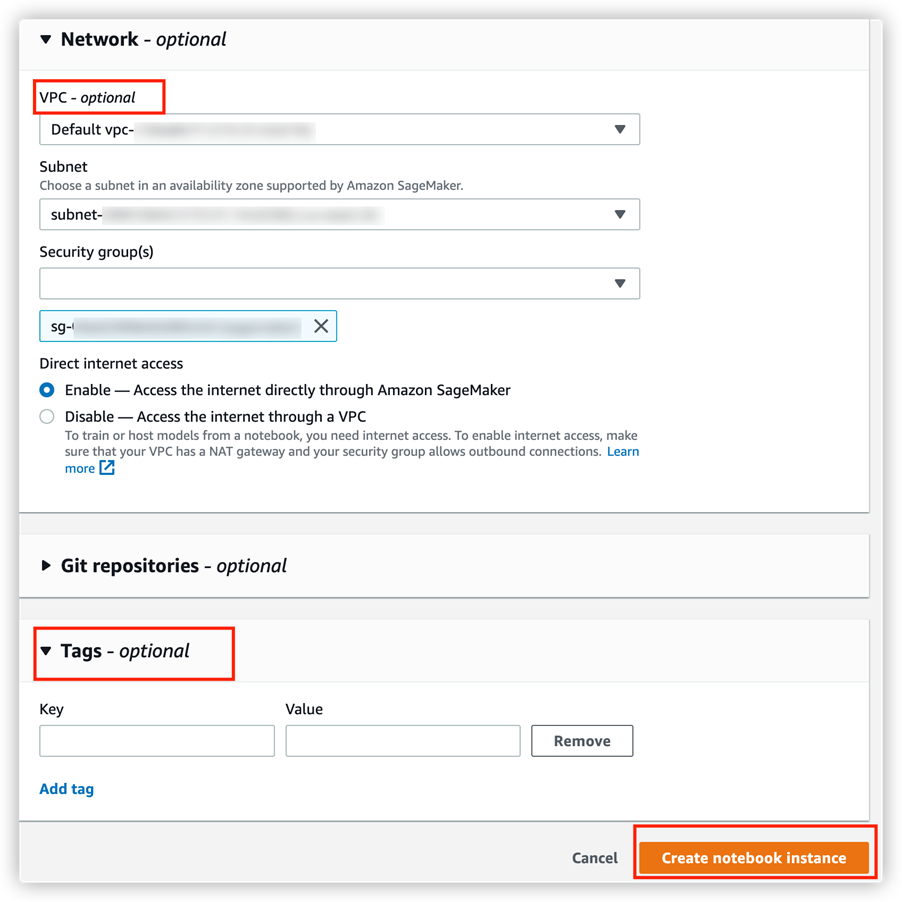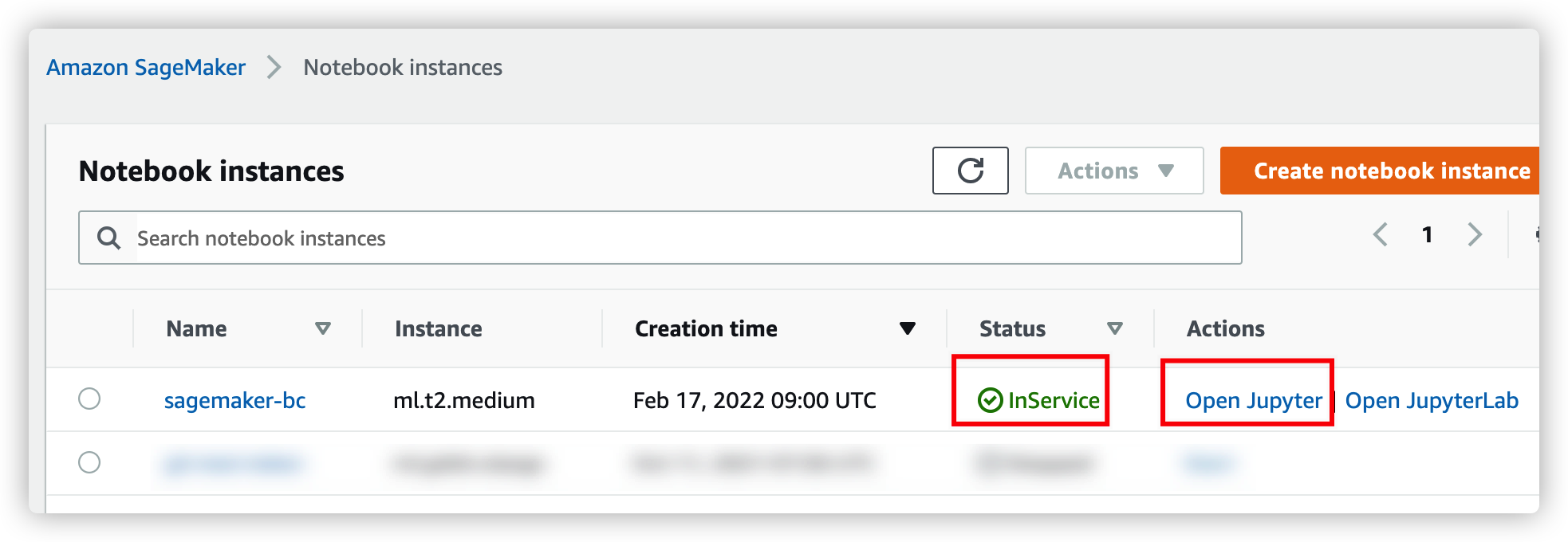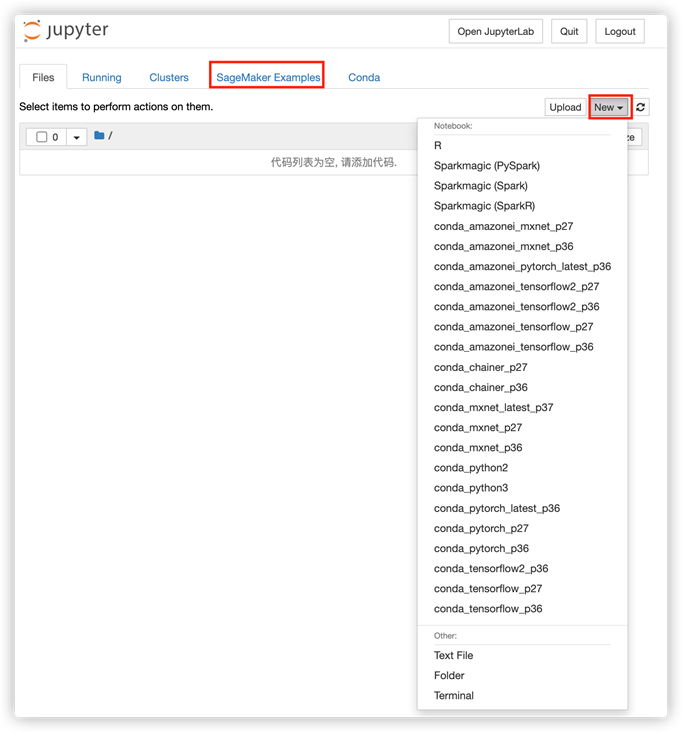## 3、数据集探索

3) bank-full.csv包含所有的样本，每个样本17个特征，按日期排序（旧版本的数据集，特征较少）。

4) bank.csv中有10%的样本，每个样本17个特征，从3）中所述数据集中随机采样。

### 3.1数据集下载

``````!wget -N https://archive.ics.uci.edu/ml/machine-learning-databases/00222/bank-additional.zip

### 3.2数据集一览

``````import numpy as np  # For matrix operations and numerical processing
import pandas as pd  # For munging tabular data
import os

pd.set_option("display.max_columns", 500)  # Make sure we can see all of the columns
pd.set_option("display.max_rows", 50)  # Keep the output on one page
data
``````
`得到如下数据预览图：`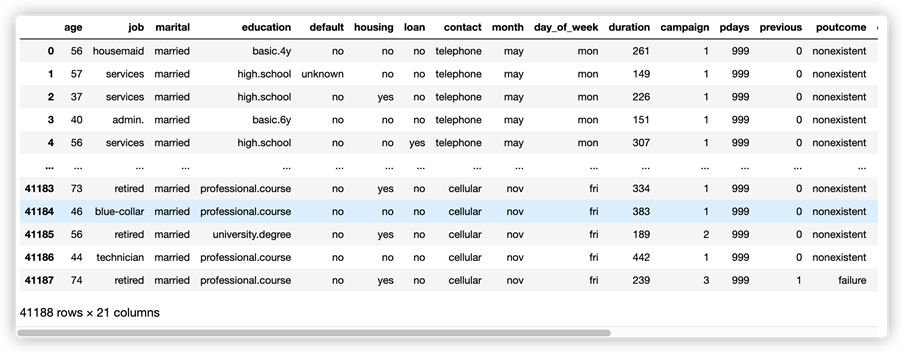• 我们有40K多一点的样本（客户记录），每个样本有20个特征；
• 这些特征的类型有些数字类型，有些分类类型；
• 这些数据似乎是按时间和联系方式排序的；

 特征名称 解释 字段类型 取值示例 人口统计相关特征 age 客户的年龄 数值 23 job 工作类型 分类 ‘admin.’，’services’，… marital 婚姻状况 分类 ‘married’, ‘single’，… education 教育水平 分类 ‘basic.4y’，’high.school’，… 过去与该客户有关的活动 default 有信用违约吗 分类 ‘no’，’unknown’，… housing 有房贷吗 分类 ‘no’，’yes’，… loan 有个人贷款吗 分类 ‘no’，’yes’，… 过去的营销时联系情况 contact 联系方式 分类 ‘cellular’, ‘telephone’, … month 上次联系时月份 分类 ‘may’, ‘nov’, … day_of_week 上次联系时周几 分类 ‘mon’, ‘fri’, … duration 上次联系时通话持续时间，以秒为单位（数字）。重要提示：该属性高度影响输出目标(例如，如果duration=0，用户将不知道该活动，那么自然不会参与，即y=’no’)。同样，在打完电话后，用户是否会参与活动将是明确的，即y将是确定的。然而，在打电话之前，这次电话的持续时间是无法获知的，因此建模时应放弃这种输入。 数值 11 营销活动信息 campaign 在此活动期间和为此客户的联系次数 数值 4 pdays 从上一个营销活动中最后一次联系该客户到现在经过的天数 数值 90 previous 在此活动之前和为此客户的联系次数 数值 7 poutcome 上一次营销活动的结果 分类 ‘nonexistent’,’success’, … 外部环境因素 emp.var.rate 就业变化率 – 季度指标 数值 1.1 cons.price.idx 消费者价格指数 – 月度指标 数值 93.994 cons.conf.idx 消费者信心指数 – 月度指标 数值 -36.4 euribor3m Euribor 3 个月利率 – 每日指标 数值 4.857 nr.employed 员工人数 – 季度指标 数值 5191.0 目标变量（标签） y 客户是否定期存款 分类 ‘yes’,’no’

### 3.3 数据预处理

（1）处理缺失值：一些机器学习算法能够处理缺失值，但大多数并不能自动处理。方法包括：

• 删除具有缺失值的样本：如果只有一小部分样本具有不完整的信息，则此方法效果很好。
• 删除具有缺失值的特征：如果有少量特征具有大量缺失值，则此方法效果很好。
• 估算缺失值：关于这个主题有人已经写了本书，但常见的选择是用该列的非缺失值的众数或平均值替换缺失值。

（2）将分类转换为数字表示：最常见的方法是一种独热编码（one hot encoding），其方法是使用N位状态寄存器来对N个状态进行编码，每个状态都由他独立的寄存器位，并且在任意时候，其中只有一位有效。

（3）异常分布的数据：虽然对于像梯度提升树这样的非线性模型影响有限，但像回归这样的模型在输入高度倾斜的数据时会产生非常不准确的估计。在某些情况下，简单地取特征的自然对数就足以产生更正态分布的数据。在其他情况下，可以将值分桶到离散范围。然后，这些分桶后的数据可以被视为分类类型的特征，并应用在独热编码中。

（4）处理更复杂的数据类型：以不同的粒度对图像、文本或数据进行处理。

``````data["no_previous_contact"] = np.where(
data["pdays"] == 999, 1, 0
)  # Indicator variable to capture when pdays takes a value of 999
data["not_working"] = np.where(
np.in1d(data["job"], ["student", "retired", "unemployed"]), 1, 0
)  # Indicator for individuals not actively employed
model_data = pd.get_dummies(data)  # Convert categorical variables to sets of indicators
model_data``````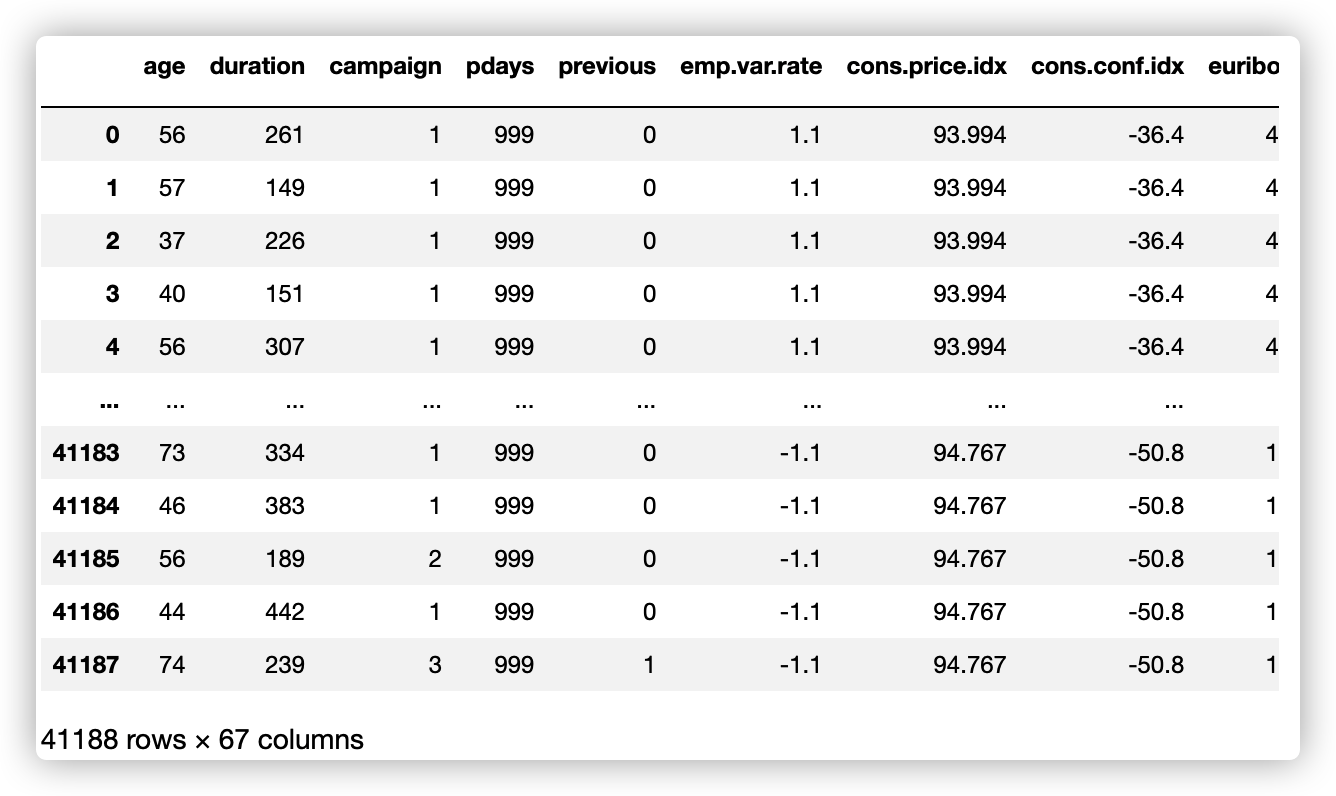``````model_data = model_data.drop(
["duration", "emp.var.rate", "cons.price.idx", "cons.conf.idx", "euribor3m", "nr.employed"], axis=1)
model_data = model_data.drop(["y_no"], axis=1)
model_data``````

``````train_data, test_data = np.split(
model_data.sample(frac=1, random_state=1729),
[int(0.9 * len(model_data)),],
)

train_x = train_data.iloc[:,:-1]
train_y = train_data.iloc[:,59]

test_x = test_data.iloc[:,:-1]
test_y = test_data.iloc[:,59]``````

## 4、使用XGBoost训练模型

XGBoost 是一个优化的分布式梯度提升库，它是在 Gradient Boosting 框架下实现的机器学习算法。XGBoost 提供了一种并行提升树（也称为 GBDT、GBM），可以快速准确地解决许多数据科学问题，相同的代码可以在主流的分布式环境（Hadoop、SGE、MPI）上运行，是算法工程师广泛应用的机器学习算法之一。

### 4.1 XGBoost安装：

`!pip install xgboost`

### 4.2 XGBoost Python API

XGBoost提供了多种开发语言包，这里我们继续使用Python开发语言。

``````import numpy as np
import pandas as pd
import xgboost as xgb
from sklearn.model_selection import train_test_split

# 用sklearn.cross_validation进行训练数据集划分，这里训练集和交叉验证集比例为8：2，可以自己根据需要设置
X, val_X, y, val_y = train_test_split(
train_x,
train_y,
test_size=0.2,
random_state=2022,
stratify=train_y
)

# xgb矩阵赋值
xgb_val = xgb.DMatrix(val_X, label=val_y)
xgb_train = xgb.DMatrix(X, label=y)
xgb_test = xgb.DMatrix(test_x)

# xgboost模型 #####################
params = {
'booster': 'gbtree',
'objective': 'binary:logistic',
'eval_metric': 'auc', #logloss
'gamma': 0.1,  # 用于控制是否后剪枝的参数,越大越保守，一般0.1、0.2
'max_depth': 8,  # 构建树的深度，越大越容易过拟合
'alpha': 0,   # L1正则化系数
'lambda': 10,  # 控制模型复杂度的权重值的L2正则化项参数，参数越大，模型越不容易过拟合
'subsample': 0.7,  # 随机采样训练样本
'colsample_bytree': 0.5,  # 生成树时进行的列采样
'min_child_weight': 3,
# 这个参数默认是 1，是每个叶子里面 h 的和至少是多少，对正负样本不均衡时的 0-1 分类而言
# ，假设 h 在 0.01 附近，min_child_weight 为 1 意味着叶子节点中最少需要包含 100 个样本。
# 这个参数非常影响结果，控制叶子节点中二阶导的和的最小值，该参数值越小，越容易 overfitting。
'silent': 0,  # 设置成1则没有运行信息输出，最好是设置为0.
'eta': 0.03,  # 如同学习率
'seed': 1000,
'nthread': -1,  # cpu 线程数
'missing': 1,
'scale_pos_weight': (np.sum(y==0)/np.sum(y==1))  # 用来处理正负样本不均衡的问题,通常取：sum(negative cases) / sum(positive cases)
}

plst = list(params.items())
num_rounds = 500  # 迭代次数
watchlist = [(xgb_train, 'train'), (xgb_val, 'val')]

# 训练模型并保存
# early_stopping_rounds 当设置的迭代次数较大时，early_stopping_rounds 可在一定的迭代次数内准确率没有提升就停止训练
model = xgb.train(plst, xgb_train, num_rounds, watchlist, early_stopping_rounds=200)
model.save_model('./xgb.model')  # 用于存储训练出的模型

preds = model.predict(xgb_test)

# 导出结果
threshold = 0.5
ypred = np.where(preds > 0.5, 1, 0)

from sklearn import metrics

print ('AUC: %.4f' % metrics.roc_auc_score(test_y,ypred))
print ('ACC: %.4f' % metrics.accuracy_score(test_y,ypred))
print ('Recall: %.4f' % metrics.recall_score(test_y,ypred))
print ('F1-score: %.4f' %metrics.f1_score(test_y,ypred))
print ('Precesion: %.4f' %metrics.precision_score(test_y,ypred))
print(metrics.confusion_matrix(test_y,ypred))``````

``````from xgboost import plot_importance
from matplotlib import pyplot as plt

plt.rcParams['figure.figsize'] = (10.0, 8.0) #

# 显示重要特征
plot_importance(model)
plt.show()``````
`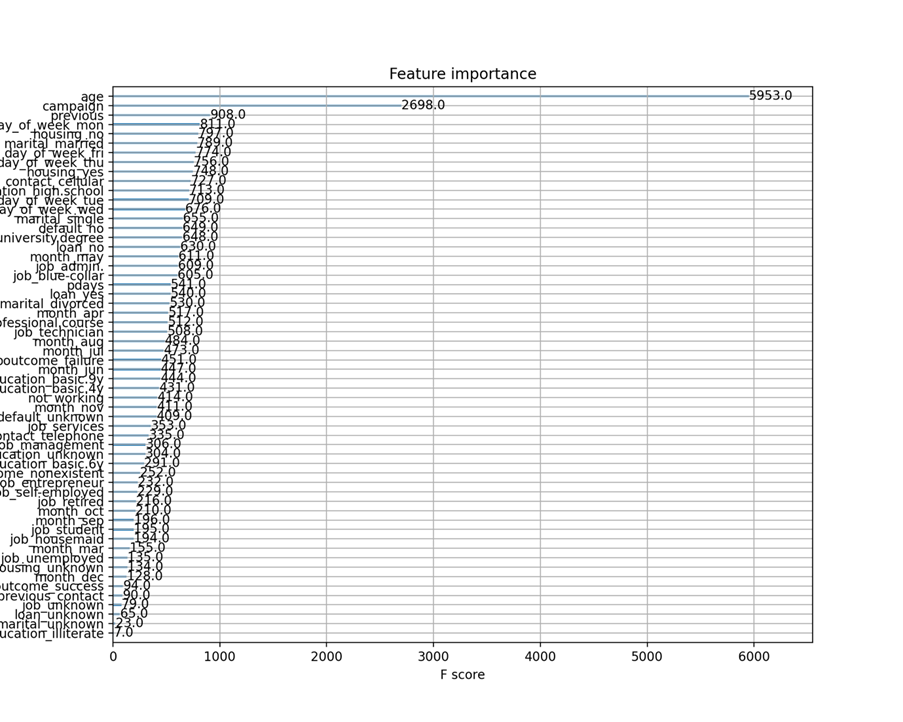`

### 4.3 XGBoost Scikit-Learn接口

XGBoost同时还提供了Scikit Learn风格的API封装，如下示例：

``````# Scikit-Learn style API
from xgboost import XGBClassifier

model = XGBClassifier(**params,n_estimators=500)
eval_set = [(val_X, val_y)]
model.fit(X, y, early_stopping_rounds=200, eval_set=eval_set, eval_metric='auc', verbose=True)

#输出概率
preds = model.predict(test_x)

# 导出结果
y_pred = [round(pred) for pred in preds]

from sklearn import metrics
print ('AUC: %.4f' % metrics.roc_auc_score(test_y,ypred))
print ('ACC: %.4f' % metrics.accuracy_score(test_y,y_pred))
print ('Recall: %.4f' % metrics.recall_score(test_y,y_pred))
print ('F1-score: %.4f' %metrics.f1_score(test_y,y_pred))
print ('Precesion: %.4f' %metrics.precision_score(test_y,y_pred))
print(metrics.confusion_matrix(test_y,y_pred))``````

## 5、使用SageMaker内置算法训练模型

SageMaker 除了提供Notebook实例之外，还提供了模型训练和模型部署等功能，算法工程师可以高效、经济的开展机器学习项目。在模型训练方面，SageMaker 提供了Training相关的API，在该API调用中，指定模型训练需要的实例类型，数量，运行环境容器镜像以及数据集在S3中的位置等参数，SageMaker将创建相应的实例资源，自动将数据集从S3下载到新建的实例资源中，并自动启动模型训练脚本，训练完成后（生成模型），自动将模型文件上传回S3，并自动终止训练实例资源，以停止计费。训练实例的计费是按秒计费，并且支持Spot实例，节省高达90%的费用。

SageMaker Training API的调用脚本可以在前面介绍的SageMaker Notebook实例上执行，此时您只需要一个小型号的实例即可，无需使用大机型，甚至可以在您的云端 EC2实例，本地个人电脑 IDE等等，并且可以使用Java，.NET等开发语言进行SageMaker Training API的调用。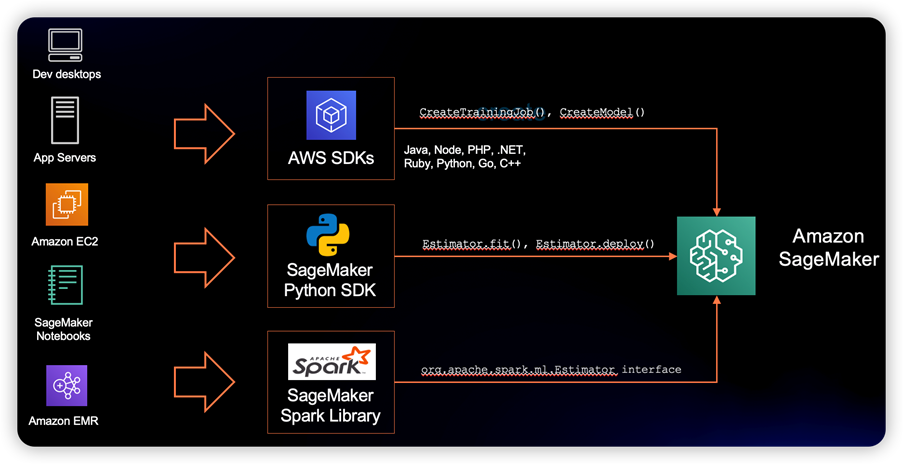### 5.1 使用SageMaker Training API开展模型训练

``````import sagemaker
import boto3

import numpy as np  # For matrix operations and numerical processing
import pandas as pd  # For munging tabular data
from time import gmtime, strftime
import os

region = boto3.Session().region_name
smclient = boto3.Session().client("sagemaker")

role = sagemaker.get_execution_role()

bucket = sagemaker.Session().default_bucket()
prefix = "sagemaker/DEMO-hpo-xgboost-dm"``````

``````data = pd.read_csv("./bank-additional/bank-additional-full.csv", sep=";")
pd.set_option("display.max_columns", 500)  # Make sure we can see all of the columns
data["no_previous_contact"] = np.where(
data["pdays"] == 999, 1, 0
)  # Indicator variable to capture when pdays takes a value of 999
data["not_working"] = np.where(
np.in1d(data["job"], ["student", "retired", "unemployed"]), 1, 0
)  # Indicator for individuals not actively employed
model_data = pd.get_dummies(data)  # Convert categorical variables to sets of indicators
model_data = model_data.drop(
["duration", "emp.var.rate", "cons.price.idx", "cons.conf.idx", "euribor3m", "nr.employed"],
axis=1,

)``````

``````train_data, validation_data, test_data = np.split(
model_data.sample(frac=1, random_state=1729),
[int(0.7 * len(model_data)), int(0.9 * len(model_data))],
)

pd.concat([train_data["y_yes"], train_data.drop(["y_no", "y_yes"], axis=1)], axis=1).to_csv(
)
pd.concat(
[validation_data["y_yes"], validation_data.drop(["y_no", "y_yes"], axis=1)], axis=1
pd.concat([test_data["y_yes"], test_data.drop(["y_no", "y_yes"], axis=1)], axis=1).to_csv(
)``````

``````boto3.Session().resource("s3").Bucket(bucket).Object(
os.path.join(prefix, "train/train.csv")
boto3.Session().resource("s3").Bucket(bucket).Object(
os.path.join(prefix, "validation/validation.csv")

from sagemaker.inputs import TrainingInput

s3_input_train = TrainingInput(
s3_data="s3://{}/{}/train".format(bucket, prefix), content_type="csv"
)
s3_input_validation = TrainingInput(
s3_data="s3://{}/{}/validation/".format(bucket, prefix), content_type="csv"
)``````

``````from sagemaker.debugger import Rule, rule_configs

rules=[
Rule.sagemaker(rule_configs.create_xgboost_report())
]

sess = sagemaker.Session()

container = sagemaker.image_uris.retrieve("xgboost", boto3.Session().region_name, "1.2-1")

xgb = sagemaker.estimator.Estimator(
container,
role,
instance_count=1,
instance_type="ml.m4.xlarge",
base_job_name="bank-dm-xgboost-report",
output_path="s3://{}/{}/output".format(bucket, prefix),
sagemaker_session=sess,
rules=rules
)

xgb.set_hyperparameters(
max_depth=5,
eta=0.2,
gamma=4,
min_child_weight=6,
subsample=0.8,
objective="binary:logistic",
num_round=500,
)

xgb.fit({"train": s3_input_train, "validation": s3_input_validation})``````

``````2022-02-18 09:16:46 Starting - Starting the training job...
2022-02-18 09:17:09 Starting - Launching requested ML instancesCreateXgboostReport: InProgress
ProfilerReport-1645175806: InProgress
......
2022-02-18 09:18:15 Starting - Preparing the instances for training...............
2022-02-18 09:21:30 Training - Training image download completed. Training in progress.
.........等等训练过程日志``````

``````2022-02-18 09:22:16 Uploading - Uploading generated training model
2022-02-18 09:22:16 Completed - Training job completed
Training seconds: 98
Billable seconds: 40
Managed Spot Training savings: 59.2%``````

### 5.2 训练任务管理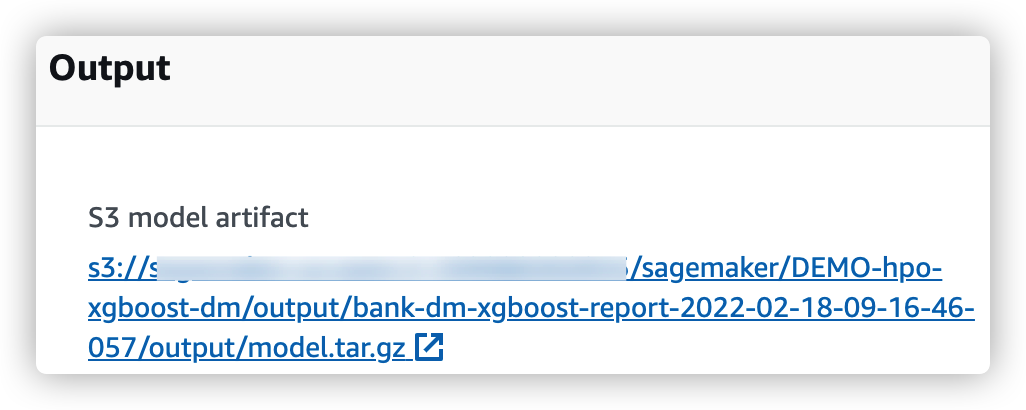### 5.3 SageMaker XGBoost训练报告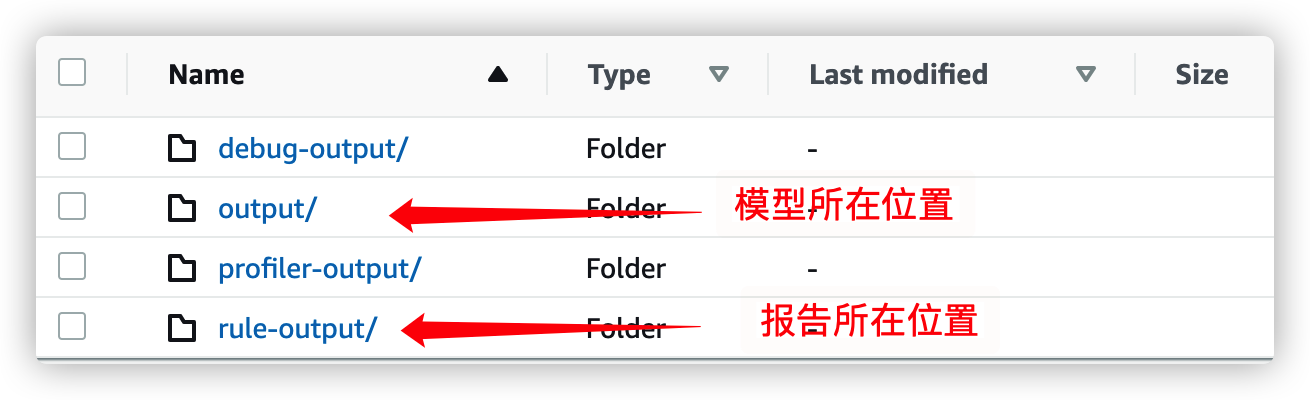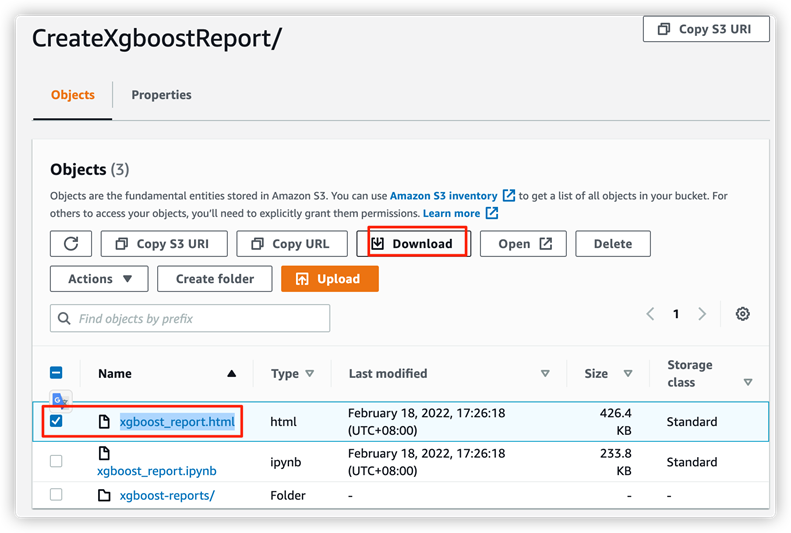## 6、使用AutoGluon训练模型

AutoGluon 是一个流行的开源易用的AutoML模型训练包，支持文本、图像和表格数据的应用场景，AutoGluon 面向 ML 初学者和专家，使您能够：

• 只需几行代码，即可为您的原始数据快速构建深度学习和传统机器学习模型原型。
• 在没有专业知识的情况下自动利用最先进的技术（在适当的情况下）。
• 自动超参数调整、模型选择/集成、架构搜索和数据处理。
• 轻松改进/调整您的定制模型和数据管道，或为您的用例定制 AutoGluon。

### 6.1 AutoGluon安装

``````# Install AutoGluon
!pip install -U setuptools wheel
!pip install -U "mxnet<2.0.0"
!pip install autogluon``````

### 6.2 使用AutoGluon Tabular训练模型

``````from autogluon.tabular import TabularDataset, TabularPredictor
label = 'y'
print("Summary of y variable: \n", ag_data[label].describe())

ag_train_data, ag_test_data = np.split(
ag_data.sample(frac=1, random_state=1729),
[int(0.9 * len(model_data)),],
)``````

``````ag_test_data_X = ag_test_data.iloc[:,:-1]
ag_test_data_y =ag_test_data.iloc[:,20]

save_path = 'agModels-predictClass'  # specifies folder to store trained models

learner_kwargs = {'ignored_columns':[["duration", "emp.var.rate", "cons.price.idx", "cons.conf.idx", "euribor3m", "nr.employed"]]}

predictor = TabularPredictor(label=label, path=save_path,
eval_metric='recall', learner_kwargs=learner_kwargs
).fit(ag_train_data)
predictor = TabularPredictor.load(save_path)  # unnecessary, just demonstrates how to load previously-trained predictor from file

ag_y_pred = predictor.predict(ag_test_data_X)
ag_y_pred_proa = predictor.predict_proba(ag_test_data_X)

print("Predictions:  \n", ag_y_pred)
perf = predictor.evaluate_predictions(y_true=ag_test_data_y, y_pred=ag_y_pred, auxiliary_metrics=True)
# perf = predictor.evaluate_predictions(y_true=ag_test_data_y, y_pred=ag_y_pred_proa, auxiliary_metrics=True) #when eval_metric='auc' in TabularPredictor()``````

## 参考资料

XGBoost Python API

XGBoot XGBClassifier

XGBoost代码示例

SageMaker Python SDK

SageMaker 内置算法示例1

SageMaker内置算法示例2

AutoGluon Quickstart

AutoGluon TabularPredictor

## 本篇作者